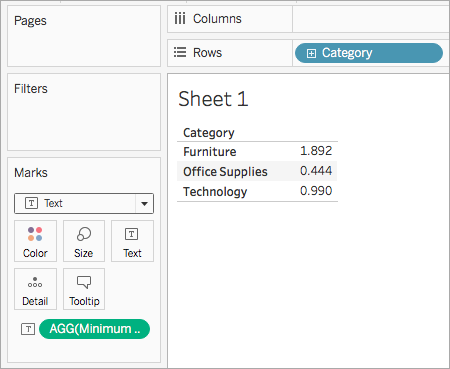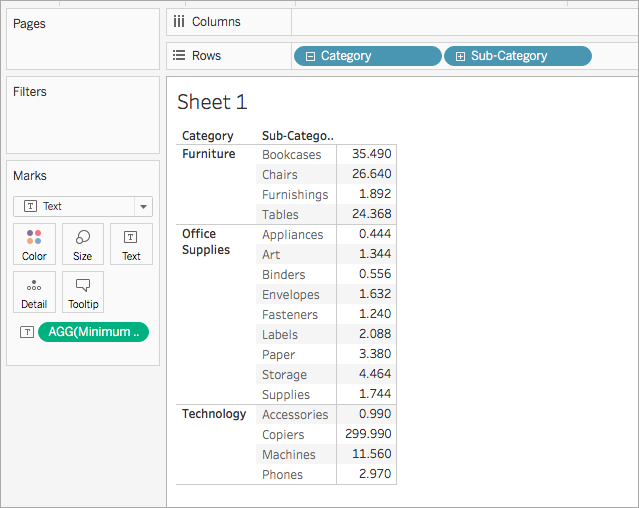This article introduces number functions and their uses in Tableau. It also demonstrates how to create a number calculation using an example.

## Why use number functions

Number functions allow you to perform computations on the data values in your fields. Number functions can only be used with fields that contain numerical values. For more information, see Data Types.

For example, you might have a field that contains values for the variance in your budget, titled Budget Variance. One of those values might be -7. You can use the ABS function to return the absolute value of that number, and all the other numbers in that field.

The calculation might look something like this:

`ABS[Budget Variance]`

Therefore, ABS(-7) = 7.

## Number functions available in Tableau

#### Description

ABS

`ABS(number)`

Returns the absolute value of the given number.

Examples:

`ABS(-7) = 7`
`ABS([Budget Variance])`

The second example returns the absolute value for all the numbers contained in the `Budget Variance` field.

ACOS

`ACOS(number)`

Returns the arc cosine of the given number. The result is in radians.

Example:

```ACOS(-1) = 3.14159265358979```

ASIN

`ASIN(number)`

Returns the arc sine of a given number. The result is in radians.

Example:

`ASIN(1) = 1.5707963267949`

ATAN

`ATAN(number)`

Returns the arc tangent of a given number. The result is in radians.

Example:

```ATAN(180) = 1.5652408283942```

ATAN2

`ATAN2(y number, x number)`

Returns the arc tangent of two given numbers (x and y). The result is in radians.

Example:

`ATAN2(2, 1) = 1.10714871779409`

CEILING

`CEILING(number)`

Rounds a number to the nearest integer of equal or greater value.

Example:

`CEILING(3.1415) = 4`

#### Availability by data source:

 Data Source Support Microsoft Access Not supported Microsoft Excel Supported Text File Supported Statistical File Supported Tableau Server Supported Actian Vector Not supported Amazon Aurora for MySQL Not supported Amazon EMR Hadoop Hive Supported Amazon Redshift Supported Aster Database Not supported Cloudera Hadoop Supported DataStax Enterprise Supported EXASOL Not supported Firebird Not supported Google Analytics Supported Google BigQuery Supported Google Cloud SQL Not supported Google Sheets Not supported Hortonworks Hadoop Hive Supported IBM BigInsights Not supported IBM DB2 Not supported IBM PDA (Netezza) Not supported MapR Hadoop Hive Supported MarkLogic Not supported Microsoft Analysis Services Not supported Microsoft PowerPivot Not supported Microsoft SQL Server Supported MySQL Not supported Oracle Not supported Oracle Essbase Not supported Actian Matrix (ParAccel) Not supported Pivotal Greenplum Not supported PostgreSQL Not supported Progress OpenEdge Not supported Salesforce Supported SAP HANA Not supported SAP Sybase ASE Not supported SAP Sybase IQ Not supported Spark SQL Supported Splunk Not supported Teradata Not supported Teradata OLAP Connector Not supported Vertica Not supported

COS

`COS(number)`

Returns the cosine of an angle. Specify the angle in radians.

Example:

`COS(PI( ) /4) = 0.707106781186548`

COT

`COT(number)`

Returns the cotangent of an angle. Specify the angle in radians.

Example:

`COT(PI( ) /4) = 1`

DEGREES

`DEGREES(number)`

Converts a given number in radians to degrees.

Example:

`DEGREES(PI( )/4) = 45.0`

DIV

DIV(integer1, integer2)

Returns the integer part of a division operation, in which integer1 is divided by integer2.

Example:

`DIV(11,2) = 5`

EXP

`EXP(number)`

Returns e raised to the power of the given number.

Examples:

`EXP(2) = 7.389`
```EXP(-[Growth Rate]*[Time])```

FLOOR

`FLOOR(number)`

Rounds a number to the nearest integer of equal or lesser value.

Example:

`FLOOR(3.1415) = 3`

#### Availability by data source:

 Data Source Support Microsoft Access Not supported Microsoft Excel Supported Text File Supported Statistical File Supported Tableau Server Supported Actian Vector Not supported Amazon Aurora for MySQL Not supported Amazon EMR Hadoop Hive Supported Amazon Redshift Not supported Aster Database Not supported Cloudera Hadoop Supported DataStax Enterprise Supported EXASOL Not supported Firebird Not supported Google Analytics Supported Google BigQuery Supported Google Cloud SQL Not supported Hortonworks Hadoop Hive Supported IBM BigInsights Not supported IBM DB2 Not supported IBM Netezza Not supported MapR Hadoop Hive Supported MarkLogic Not supported Microsoft Analysis Services Not supported Microsoft PowerPivot Not supported Microsoft SQL Server Supported MySQL Not supported Oracle Not supported Oracle Essbase Not supported ParAccel Not supported Pivotal Greenplum Not supported PostgreSQL Not supported Progress OpenEdge Not supported Salesforce Supported SAP HANA Not supported SAP Sybase ASE Not supported SAP Sybase IQ Not supported Spark SQL Supported Splunk Not supported Teradata Not supported Teradata OLAP Connector Not supported Vertica Not supported

HEXBINX

`HEXBINX(number, number)`

Maps an x, y coordinate to the x-coordinate of the nearest hexagonal bin. The bins have side length 1, so the inputs may need to be scaled appropriately.

HEXBINX and HEXBINY are binning and plotting functions for hexagonal bins. Hexagonal bins are an efficient and elegant option for visualising data in an x/y plane such as a map. Because the bins are hexagonal, each bin closely approximates a circle and minimises variation in the distance from the data point to the centre of the bin. This makes the clustering both more accurate and informative.

Example:

`HEXBINX([Longitude], [Latitude])`

HEXBINY

`HEXBINY(number, number)`

Maps an x, y coordinate to the y-coordinate of the nearest hexagonal bin. The bins have side length 1, so the inputs may need to be scaled appropriately.

Example:

`HEXBINY([Longitude], [Latitude])`

LN

`LN(number)`

Returns the natural logarithm of a number. Returns `Null` if number is less than or equal to 0.

LOG

`LOG(number [, base])`

Returns the logarithm of a number for the given base. If the base value is omitted, base 10 is used.

MAX

`MAX(number, number)`

Returns the maximum of the two arguments, which must be of the same type. Returns `Null` if either argument is `Null`. `MAX` can also be applied to a single field in an aggregate calculation.

Examples:

`MAX(4,7)`
`MAX(Sales,Profit)`
```MAX([First Name],[Last Name])```

MIN

`MIN(number, number)`

Returns the minimum of the two arguments, which must be of the same type. Returns `Null` if either argument is `Null`. `MIN` can also be applied to a single field in an aggregate calculation.

Examples:

`MIN(4,7)`
`MIN(Sales,Profit)`
```MIN([First Name],[Last Name])```

PI

`PI( )`

Returns the numeric constant pi: 3.14159.

POWER

`POWER(number, power)`

Raises the number to the specified power.

Examples:

```POWER(5,2) = 52 = 25```
`POWER(Temperature, 2)`

You can also use the ^ symbol:

```5^2 = POWER(5,2) = 25```

`RADIANS(number)`

Converts the given number from degrees to radians.

Example:

`RADIANS(180) = 3.14159`

ROUND

`ROUND(number, [decimals])`

Rounds numbers to a specified number of digits. The `decimals` argument specifies how many decimal points of precision to include in the final result. If `decimals` is omitted, `number` is rounded to the nearest integer.

Example:

This example rounds every `Sales` value to an integer:

`ROUND(Sales)`

Some databases, such as SQL Server, allow specification of a negative `length`, where -1 rounds `number` to 10's, -2 rounds to 100's and so on. This is not true of all databases. For example, it is not true of Excel or Access.

Note: because ROUND may run into issues due to the underlying floating point representation of numbers – such as 9.405 rounding to 9.40 – it may be preferable to format the number to the desired number of decimal points rather than rounding. Formatting 9.405 to two decimal places will yield the expected 9.41.

SIGN

`SIGN(number)`

Returns the sign of a number: The possible return values are -1 if the number is negative, 0 if the number is zero, or 1 if the number is positive.

Example:

If the average of the profit field is negative, then

```SIGN(AVG(Profit)) = -1```

SIN

`SIN(number)`

Returns the sine of an angle. Specify the angle in radians.

Examples:

```SIN(0) = 1.0```
`SIN(PI( )/4) = 0.707106781186548`

SQRT

`SQRT(number)`

Returns the square root of a number.

Example:

`SQRT(25) = 5`

SQUARE

`SQUARE(number)`

Returns the square of a number.

Example:

`SQUARE(5) = 25`

TAN

`TAN(number)`

Returns the tangent of an angle. Specify the angle in radians.

Example:

```TAN(PI ( )/4) = 1.0```

ZN

`ZN(expression)`

Returns the expression if it is not null, otherwise returns zero. Use this function to use zero values instead of null values.

Example:

`ZN([Profit]) = [Profit]`

## Create a number calculation

Follow along with the steps below to learn how to create a number calculation.

1. In Tableau Desktop, connect to the Sample - Superstore saved data source, which comes with Tableau.

2. Navigate to a worksheet and select Analysis > Create Calculated Field.

3. In the calculation editor that opens, do the following:

• Name the calculated field Minimum Sales transaction

• Enter the following formula:

`MIN(Sales)`

• When finished, click OK.

The new number calculation appears under Measures in the Data pane. Just like your other fields, you can use it in one or more visualisations.

When Minimum Sales is placed on Text on the Marks card in the worksheet, its name is changed to AGG(Minimum Sales), which indicates that it cannot be aggregated any further, since it is already aggregated down to the lowest level of detail (the smallest sales value for all records).

This example shows the minimum sales per category.When subcategory is brought into the view, the minimum sales for each subcategory are shown.